Exterior Layer Drawing Object (red line) defines the outline for the processing of Unit Layer Geometry (blue line).

Valid Unit Layer Geometries

Invalid Unit Layer Geometries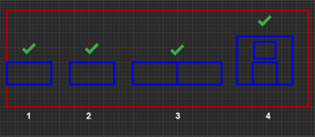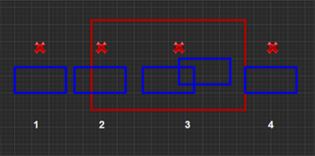1. The edge of the Unit Layer Geometry overlaps the edge of the Exterior Layer Object.
2. The Unit Layer Geometry is inside the Exterior Layer Object.
3. The edges of the Unit Layer Geometries overlaps.
4. A Geometry is completely inside another Geometry.
1. The Unit Layer Geometry is completely outside the Exterior Layer Object.
2. The Unit Layer Geometry partially overlaps the Exterior Layer Object.
3. Partially overlapping Unit Layer Geometries.
4. The edge of Unit Layer Geometry overlaps the edge of Exterior Layer, but the geometry is outside the Exterior Layer Object.

## Other examples of valid and invalid geometries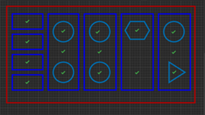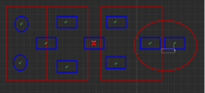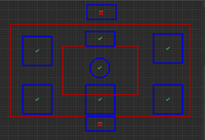Also refer the Azure Maps documentation, chapter Drawing conversion errors and warnings: https://learn.microsoft.com/en-us/azure/azure-maps/drawing-conversion-error-codes